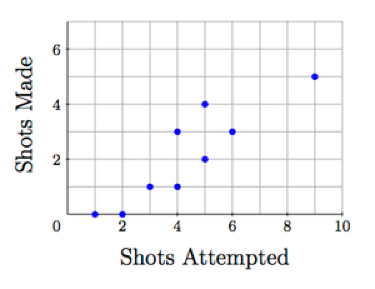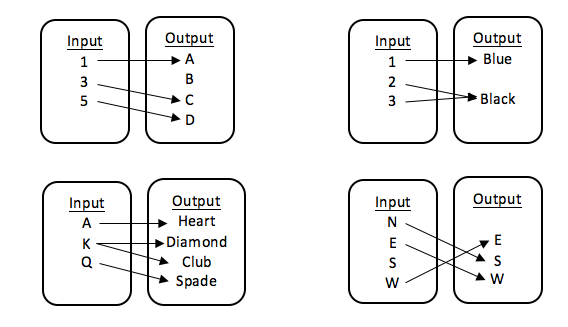# Functions

## Objective

Define and identify functions.

## Common Core Standards

### Core Standards

?

• 8.F.A.1 — Understand that a function is a rule that assigns to each input exactly one output. The graph of a function is the set of ordered pairs consisting of an input and the corresponding output. Function notation is not required in Grade 8.

## Criteria for Success

?

1. Define a function as a relationship between two sets in which every input has exactly one output.
2. Create input/output tables to represent relationships.
3. Determine if input/output tables and relationships are examples of functions.
4. Identify examples of non-functions in which either an input does not have an output or an input has more than one output.

## Tips for Teachers

?

• The following materials are needed for this lesson: individual whiteboards and dry-erase markers OR cut-out cards with the letters A, B, C, and D on them (one set of four cards per student).
• This lesson introduces students to the concept of a function to describe a relationship. Though they have worked with functions prior to eighth grade with equations and proportional relationships, this is the first time they will formally define it. Through this unit and the next unit, students will explore functions in-depth; this lesson provides the basic definition of a function as a relationship of inputs and outputs where every input has exactly one output.

#### Fishtank Plus

• Problem Set
• Student Handout Editor
• Vocabulary Package

## Anchor Problems

?

### Problem 1

Give students small whiteboards and dry-erase markers, or give each student a set of four cards labeled A, B, C, and D.

For each question you hear, write or raise the letter that corresponds to your answer.

Question #1: How many siblings do you have?

• If you have no siblings, raise the letter A.
• If you have 1 sibling, raise the letter B.
• If you have 2 siblings, raise the letter C.
• If you have 3 or more siblings, raise the letter D.

Question #2: How long did it take you to get to school today?

• If it took you fewer than 15 minutes to get to school today, raise the letter B.
• If it took you 15 minutes or more to get to school today, raise the letter D.

Question #3: What grade are you in?

• If you are in 6th grade, raise the letter A.
• If you are in 7th grade, raise the letter B.
• If you are in 8th grade, raise the letter C.
• If you are in any other grade, raise the letter D.

Question 4:

• If you are wearing blue today, raise the letter A.
• If you are wearing white today, raise the letter B.
• If you are wearing red today, raise the letter C.
• If you are wearing brown today, raise the letter D.

Question 5:

• If you were born in the summer, raise the letter A.
• If you were born in the spring, raise the letter B.

#### References

If Functions Are Aspirin, Then How Do You Create The Headache? by Dan Meyer is made available at dy/dan under the CC BY 4.0 license. Accessed Oct. 26, 2017, 7:55 p.m..

Modified by The Match Foundation, Inc.

### Problem 2

For each situation below, fill in the missing information in the tables. Then determine if each chart represents a function.

1. A parking meter takes only dimes, and each dime is worth 6 minutes on the meter.
 Number of Dimes Minutes of Parking 2 3 7

 Minutes of Parking Number of Dimes 0 12 54
1. Each point on the graph below shows how many shots a player on a basketball team took and made in the first half of a game.Shots Attempted Shots Made 2 4 7

 Shots Made Shots Attempted 1 4 6

#### References

Illustrative Mathematics Introducing FunctionsPart a.i and a.ii

Introducing Functions, accessed on Oct. 26, 2017, 7:57 p.m., is licensed by Illustrative Mathematics under either the CC BY 4.0 or CC BY-NC-SA 4.0. For further information, contact Illustrative Mathematics.

### Problem 3

Create an input/output table for each rule below.

1. Input any number. Add 2, then square the number.
2. Input any number. If it is greater than or equal to 6, then record a 0; if it less than or equal to 6, then record a 1.

## Problem Set

?The following resources include problems and activities aligned to the objective of the lesson that can be used to create your own problem set.

• Problems similar to the Target Task, where students are given tables or input/output maps and determine if they are functions